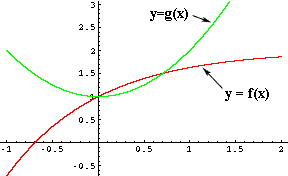Quandaries and Queries Name: benjamin Who is asking: Student Level: Secondary Question: How do I find the points of intersection of the two functions: 1) y = 2 - (e^-x) 2) y = 1 + (x^2) I know that I have to set them equal to each other, but I can't solve for x. Please help :-) Hi Benjamin, Setting them equal gives 2 - e-x = 1 + x2 1 - x2 = ex At this point, like you, I see no technique to solve for x. All I can do is use my number sense and try some values of x. This yields an immediate result since the fact that e0 = 1 shows that x = 0 is a solution. A harder question is "Is there another solution?" To do this I used some computer software to plot the two functions f(x) = 2 - e-x , and g(x) = 1 + x2From the plot I can see that x = 0 is a solution, but also that there is another solution at approximately x = 0.7. My calculator gives f(0.7) =  1.50341 and g(0.7) = 1.49, so they are not equal, but close. The best I can do now is improve my approximation. Try x = 0.71 and see what you get. Penny Go to Math Central